## Computing R.M.S. Error

RMS error measures the differences between values predicted by a model or an estimator and the values actually observed.

### Learning Objectives

Define and compute root-mean-square error.

### Key Takeaways

#### Key Points

• These individual differences are called residuals when the calculations are performed over the data sample that was used for estimation, and are called prediction errors when computed out-of-sample.
• The differences between values occur because of randomness or because the estimator doesn’t account for information that could produce a more accurate estimate.
• RMS error serves to aggregate the magnitudes of the errors in predictions for various times into a single measure of predictive power.
• In terms of a regression line, the error for the differing values is simply the distance of a point above or below the line.
• In general, about 68% of points on a scatter diagram are within one RMS error of the regression line, and about 95% are within two.

#### Key Terms

• root-mean-square error: (RMS error) A frequently used measure of the differences between values predicted by a model or an estimator and the values actually observed.

Root- mean -square (RMS) error, also known as RMS deviation, is a frequently used measure of the differences between values predicted by a model or an estimator and the values actually observed. These individual differences are called residuals when the calculations are performed over the data sample that was used for estimation, and are called prediction errors when computed out-of-sample. The differences between values occur because of randomness or because the estimator doesn’t account for information that could produce a more accurate estimate.

Root-mean-square error serves to aggregate the magnitudes of the errors in predictions for various times into a single measure of predictive power. It is also a good measure of accuracy, but only to compare forecasting errors of different models for a particular variable and not between variables, as it is scale-dependent.

RMS error is the square root of mean squared error (MSE), which is a risk function corresponding to the expected value of the squared error loss or quadratic loss. MSE measures the average of the squares of the “errors. ” The MSE is the second moment (about the origin) of the error, and thus incorporates both the variance of the estimator and its bias. For an unbiased estimator, the MSE is the variance of the estimator. Like the variance, MSE has the same units of measurement as the square of the quantity being estimated.

### Computing MSE and RMSE

If $\hat{\text{Y} }$ is a vector of $\text{n}$ predictions, and $\text{Y}$ is the vector of the true values, then the (estimated) MSE of the predictor is as given as the formula:

$\displaystyle \text{MSE} = \frac{1}{\text{n}} \sum_{\text{i}=1}^\text{n} \left(\hat{\text{Y}_\text{i}} - \text{Y}_\text{i}\right)^2$

This is a known, computed quantity given a particular sample (and hence is sample-dependent). RMS error is simply the square root of the resulting MSE quantity.

### RMS Error for the Regression Line

In terms of a regression line, the error for the differing values is simply the distance of a point above or below the line. We can find the general size of these errors by taking the RMS size for them:

$\displaystyle \sqrt { \frac { { \left( \text{error}\ 1 \right) }^{ 2 }+{ \left(\text{error}\ 2 \right) }^{ 2 }+\cdots +{ \left( \text{error \text{n}} \right) }^{ 2 } }{ \text{n} } }$.

This calculation results in the RMS error of the regression line, which tells us how far above or below the line points typically are. In general, about 68% of points on a scatter diagram are within one RMS error of the regression line, and about 95% are within two. This is known as the 68%-95% rule.

## Plotting the Residuals

The residual plot illustrates how far away each of the values on the graph is from the expected value (the value on the line).

### Learning Objectives

Differentiate between scatter and residual plots, and between errors and residuals

### Key Takeaways

#### Key Points

• The sum of the residuals within a random sample is necessarily zero, and thus the residuals are necessarily not independent.
• The average of the residuals is always equal to zero; therefore, the standard deviation of the residuals is equal to the RMS error of the regression line.
• We see heteroscedasticity in a resitual plot as the difference in the scatter of the residuals for different ranges of values of the independent variable.

#### Key Terms

• scatter plot: A type of display using Cartesian coordinates to display values for two variables for a set of data.
• residual: The difference between the observed value and the estimated function value.
• heteroscedasticity: The property of a series of random variables of not every variable having the same finite variance.

### Errors Versus Residuals

Statistical errors and residuals are two closely related and easily confused measures of the deviation of an observed value of an element of a statistical sample from its “theoretical value. ” The error of an observed value is the deviation of the observed value from the (unobservable) true function value, while the residual of an observed value is the difference between the observed value and the estimated function value.

A statistical error is the amount by which an observation differs from its expected value, the latter being based on the whole population from which the statistical unit was chosen randomly. For example, if the mean height in a population of 21-year-old men is 5′ 8″, and one randomly chosen man is 5′ 10″ tall, then the “error” is 2 inches. If the randomly chosen man is 5′ 6″ tall, then the “error” is $-2$ inches. The expected value, being the mean of the entire population, is typically unobservable, and hence the statistical error cannot be observed either.

A residual (or fitting error), on the other hand, is an observable estimate of the unobservable statistical error. Consider the previous example with men’s heights and suppose we have a random sample of $\text{n}$ people. The sample mean could serve as a good estimator of the population mean, and we would have the following:

The difference between the height of each man in the sample and the unobservable population mean is a statistical error, whereas the difference between the height of each man in the sample and the observable sample mean is a residual.

Note that the sum of the residuals within a random sample is necessarily zero, and thus the residuals are necessarily not independent. The statistical errors on the other hand are independent, and their sum within the random sample is almost surely not zero.

### Residual Plots

In scatter plots we typically plot an $\text{x}$-value and a $\text{y}$-value. To create a residual plot, we simply plot an $\text{x}$-value and a residual value. The residual plot illustrates how far away each of the values on the graph is from the expected value (the value on the line).

The average of the residuals is always equal to zero; therefore, the standard deviation of the residuals is equal to the RMS error of the regression line.

As an example, consider the figure depicting the number of drunk driving fatalities in 2006 and 2009 for various states: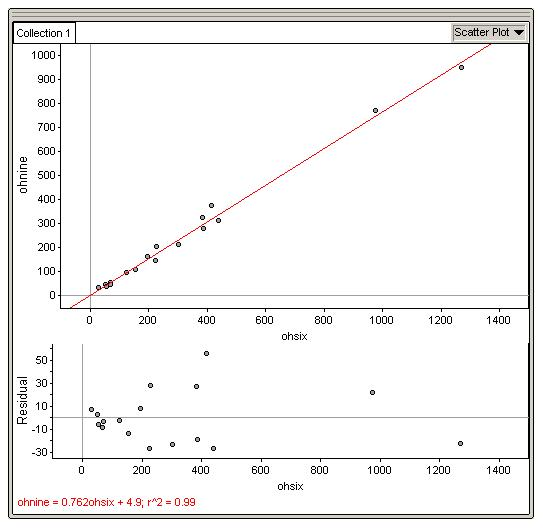Residual Plot: This figure shows a scatter plot, and corresponding residual plot, of the number of drunk driving fatalities in 2006 ($\text{x}$-value) and 2009 ($\text{y}$-value)

The relationship between the number of drunk driving fatalities in 2006 and 2009 is very strong, positive, and linear with an $\text{r}^2$ (coefficient of determination) value of 0.99. The high $\text{r}^2$ value provides evidence that we can use the linear regression model to accurately predict the number of drunk driving fatalities that will be seen in 2009 after a span of 4 years.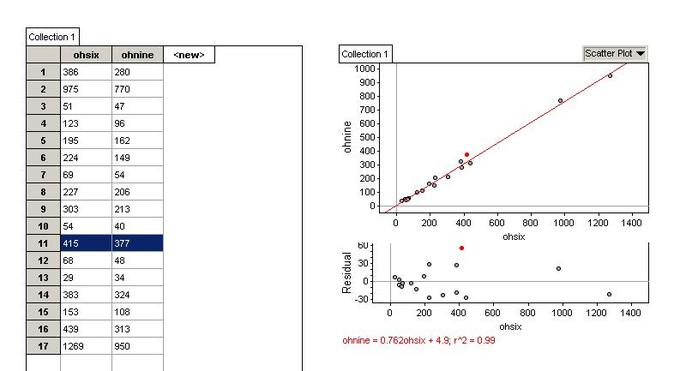High Residual: These images depict the highest residual in our example.

Considering the above figure, we see that the high residual dot on the residual plot suggests that the number of drunk driving fatalities that actually occurred in this particular state in 2009 was higher than we expected it would be after the 4 year span, based on the linear regression model. So, based on the linear regression model, for a 2006 value of 415 drunk driving fatalities we would expect the number of drunk driving fatalities in 2009 to be lower than 377. Therefore, the number of fatalities was not lowered as much as we expected they would be, based on the model.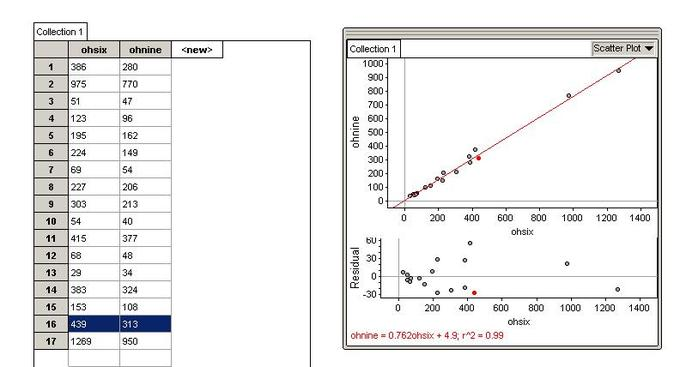Low Residual: These images depict the lowest residual in our example.

Considering the above figure, we see that the low residual plot suggests that the actual number of drunk driving fatalities in this particular state in 2009 was lower than we would have expected it to be after the 4 year span, based on the linear regression model. So, based on the linear regression model, for a 2006 value of 439 drunk driving fatalities we would expect the number of drunk driving fatalities for 2009 to be higher than 313. Therefore, this particular state is doing an exceptional job at bringing down the number of drunk driving fatalities each year, compared to other states.

Residual plots can allow some aspects of data to be seen more easily.

• We can see nonlinearity in a residual plot when the residuals tend to be predominantly positive for some ranges of values of the independent variable and predominantly negative for other ranges.
• We see outliers in a residual plot depicted as unusually large positive or negative values.
• We see heteroscedasticity in a resitual plot as the difference in the scatter of the residuals for different ranges of values of the independent variable.

The existence of heteroscedasticity is a major concern in regression analysis because it can invalidate statistical tests of significance that assume that the modelling errors are uncorrelated and normally distributed and that their variances do not vary with the effects being modelled.

## Homogeneity and Heterogeneity

By drawing vertical strips on a scatter plot and analyzing the spread of the resulting new data sets, we are able to judge degree of homoscedasticity.

### Learning Objectives

Define, and differentiate between, homoscedasticity and heteroscedasticity.

### Key Takeaways

#### Key Points

• When drawing a vertical strip on a scatter plot, the $\text{y}$-values that fall within this strip will form a new data set, complete with a new estimated average and RMS error.
• This new data set can also be used to construct a histogram, which can subsequently be used to assess the assumption that the residuals are normally distributed.
• When various vertical strips drawn on a scatter plot, and their corresponding data sets, show a similar pattern of spread, the plot can be said to be homoscedastic (the prediction errors will be similar along the regression line ).
• A residual plot displaying homoscedasticity should appear to resemble a horizontal football.
• When a scatter plot is heteroscedastic, the prediction errors differ as we go along the regression line.

#### Key Terms

• heteroscedasticity: The property of a series of random variables of not every variable having the same finite variance.
• homoscedastic: if all random variables in a sequence or vector have the same finite variance

### Vertical Strips in a Scatter Plot

Imagine that you have a scatter plot, on top of which you draw a narrow vertical strip. The $\text{y}$-values that fall within this strip will form a new data set, complete with a new estimated average and RMS error.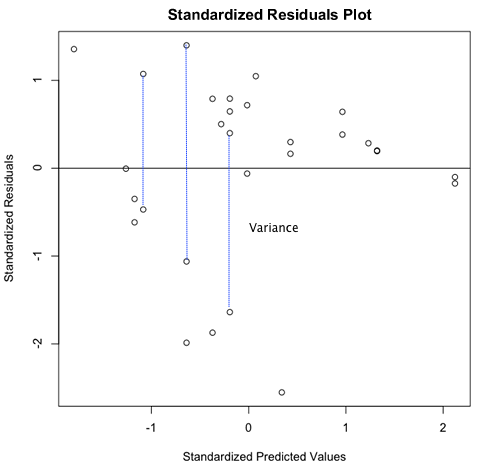Vertical Strips: Drawing vertical strips on top of a scatter plot will result in the $\text{y}$-values included in this strip forming a new data set.

This new data set can also be used to construct a histogram, which can subsequently be used to assess the assumption that the residuals are normally distributed. To the extent that the histogram matches the normal distribution, the residuals are normally distributed. This gives us an indication of how well our sample can predict a normal distribution in the population.

### Homoscedasticity Versus Heteroscedasticity

When various vertical strips drawn on a scatter plot, and their corresponding data sets, show a similar pattern of spread, the plot can be said to be homoscedastic. Another way of putting this is that the prediction errors will be similar along the regression line.

In technical terms, a data set is homoscedastic if all random variables in the sequence have the same finite variance. A residual plot displaying homoscedasticity should appear to resemble a horizontal football. The presence of this shape lets us know if we can use the regression method. The assumption of homoscedasticity simplifies mathematical and computational treatment; however, serious violations in homoscedasticity may result in overestimating the goodness of fit.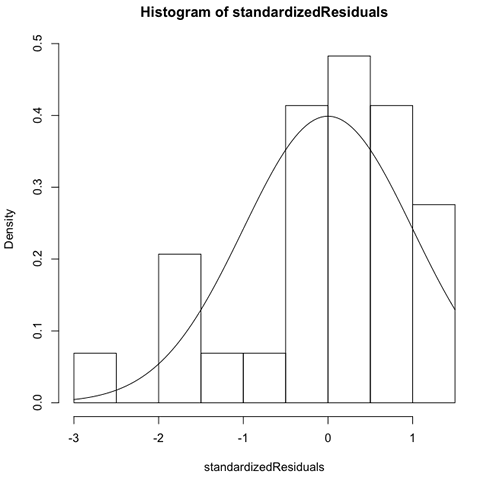Residual Histogram: To the extent that a residual histogram matches the normal distribution, the residuals are normally distributed.

In regression analysis, one assumption of the fitted model (to ensure that the least-squares estimators are each a best linear unbiased estimator of the respective population parameters) is that the standard deviations of the error terms are constant and do not depend on the $\text{x}$-value. Consequently, each probability distribution for $\text{y}$ (response variable) has the same standard deviation regardless of the $\text{x}$-value (predictor).

When a scatter plot is heteroscedastic, the prediction errors differ as we go along the regression line. In technical terms, a data set is heteroscedastic if there are sub-populations that have different variabilities from others. Here “variability” could be quantified by the variance or any other measure of statistical dispersion.

The possible existence of heteroscedasticity is a major concern in the application of regression analysis, including the analysis of variance, because the presence of heteroscedasticity can invalidate statistical tests of significance that assume that the modelling errors are uncorrelated and normally distributed and that their variances do not vary with the effects being modelled. Similarly, in testing for differences between sub-populations using a location test, some standard tests assume that variances within groups are equal.